## The local public transport authority is conducting research into commuter behaviour. A previous census indicated that the mean commuter time

Question

The local public transport authority is conducting research into commuter behaviour. A previous census indicated that the mean commuter time was 45 minutes with a standard deviation of 9.1 minutes. After some recent infrastructure upgrades and a new train timetable, the transport authority would like to test whether average commuter time has decreased. A random sample of 50 commuter times has been collected.
49.9
43.8
41
45.6
38.7
43.6
42.9
48
39.3
50.9
44.4
37.3
30.7
39.7
51.6
40.6
46.9
61.3
50.6
47.2
53
44
44.4
47.8
35.5
46.8
50.1
37.5
38.4
48.6
32.7
50.8
38.8
49
43.5
37.6
31
49.3
46.9
42.7
47.6
41.1
53.2
41.5
45.3
51.2
42.9
43.6
41.6
49.3
Conduct a hypothesis test to test whether the new changes have decreased mean commuter time.1) From the following options, select the correct null and alternate hypotheses for this test:A. H0: μ = 45, Ha: μ > 45B. H0: μ > 45, Ha: μ = 45C. H0: μ = 45, Ha: μ < 45D. H0: μ = 45, Ha: μ ≠ 45
2) Calculate the test statistic.
3) Calculate the test statistic.
4) Therefore, at a significance level of 0.05 the null hypothesis is rejectednot rejected.

in progress 0
6 months 2021-08-19T19:03:33+00:00 1 Answers 4 views 0

## Answers ( )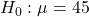and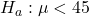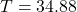The null hypothesis is not rejected

Step-by-step explanation:

Given

Random sample of 50 commuter times

Solving (a): The null and alternate hypothesis

From the question, a previous census shows that: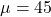and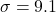This is the null hypothesis i.e.Reading further, a new test was to show whether the average has decreased.

This is the alternate hypothesis, and it is represented as:Solving (b) and (c): The test statistic (T).

This is calculated using: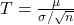In this case: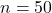andSo: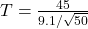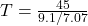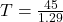Solving (c): Accept or reject null hypothesis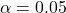First calculate the degrees of freedom (df)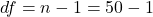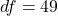From the t distribution table,

At:,andthe t score is: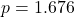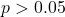So, the null hypothesis is not rejected